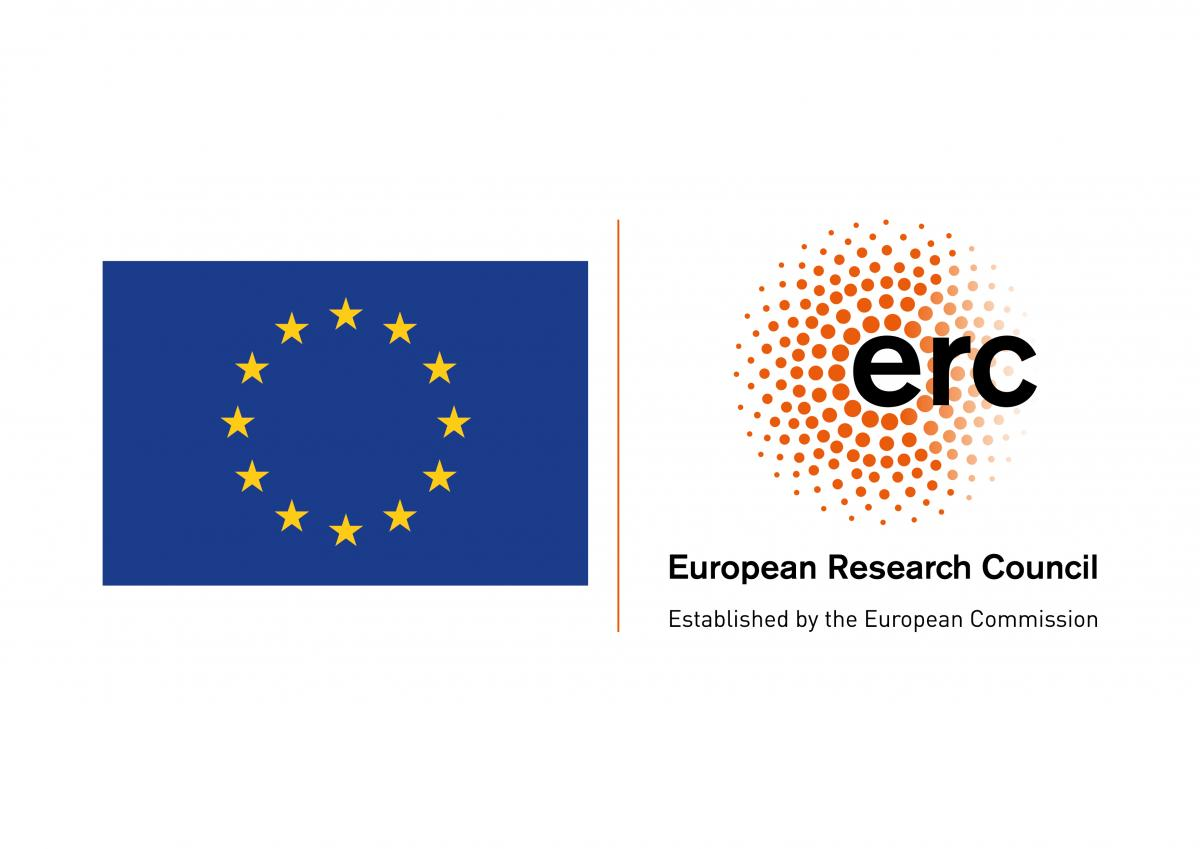## FLIRT – Fluid Flows and Irregular TransportProf. Dr. Gianluca Crippa, University of Basel, Switzerland

Several important partial differential equations (PDEs) arising in the mathematical description of physical phenomena exhibit transport features: physical quantities are advected by velocity fields that drive the dynamics of the system. This is the case for instance for the Euler equation of fluid dynamics, for conservation laws, and for kinetic equations.

An ubiquitous feature of these phenomena is their intrinsic lack of regularity. From the mathematical point of view this stems from the nonlinearity and/or nonlocality of the PDEs. Moreover, the lack of regularity also encodes actual properties of the underlying physical systems: conservation laws develop shocks (discontinuities that propagate in time), solutions to the Euler equation exhibit rough and “disordered” behaviors. This irregularity is the major difficulty in the mathematical analysis of such problems, since it prevents the use of many standard methods, foremost the classical (and powerful) theory of characteristics.

For these reasons, the study in a non smooth setting of transport and continuity equations, and of flows of ordinary differential equations, is a fundamental tool to approach challenging important questions concerning these PDEs. This project aims at establishing:

(1) deep insight into the structure of solutions of nonlinear PDEs, in particular the Euler equation and multidimensional systems of conservation laws,

(2) rigorous bounds for mixing phenomena in fluid flows, phenomena for which giving a precise mathematical formulation is extremely challenging.

The unifying factor of this proposal is that the analysis will rely on major advances in the theory of flows of ordinary differential equations in a non smooth setting, thus providing a robust formulation via characteristics for the PDEs under consideration. The guiding thread is the crucial role of geometric measure theory techniques, which are extremely efficient to describe and investigate irregular phenomena.Project acronym: FLIRT

Project: Fluid Flows and Irregular Transport

Host Institution: Universität Basel, Switzerland

Call details: Starting Grant (StG), ERC-2015-STG

Duration: 01.06.2016 – 31.05.2022